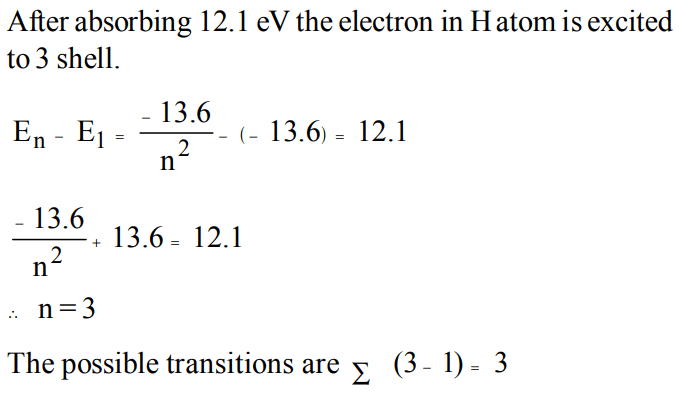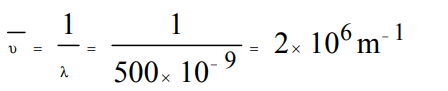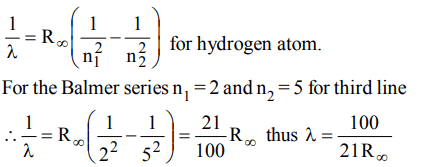## Atomic Structure Questions and Answers Part-19

1. Ionization potential of hydrogen atom is 13.6eV. Hydrogen atom in ground state are excited by monochromatic light of energy 12.1 eV. The spectral lines emitted by hydrogen according to Bohr's theory will be
a) one
b) two
c) three
d) four

Explanation:2. The wavelength of radiations emitted when electrons falls from 4th Bohr's orbit to 2nd in H atom is : $\left(R_{H}=1.09678 ×10^{-7}m^{-1}\right)$.
a) 972 nm
b) 486 nm
c) 243 nm
d) 182 nm

Explanation:3. The energy of e- in first orbit of  $He^{+}is –871.6 × 10^{-20}J$    . The energy of e- in first orbit of H is:
a) $–871.6 ×10^{-20}J$
b) $–435.8 ×10^{-20}J$
c) $– 217.9 ×10^{-20}J$
d) $–108.9 ×10^{-20}J$

Explanation:4. The wave no. of radiation of wavelength 500 nm is :
a) $5 ×10 ^{-7}/m$
b) $2 ×10 ^{7}/m$
c) $2 ×10 ^{6}/m$
d) $500 ×10 ^{-9}/m$

Explanation:5. In ground state of $Cu^{+}$. The no. of shells occupied, subshells, filled orbitals, and unpaired electrons respectively are :
a) 4, 8, 15, 0
b) 3, 6, 15, 1
c) 3, 6, 14, 0
d) 4, 7, 14, 2

Explanation: The ground state electronic configuration, of Cu+ ion is 1s2 , 2s2 , 2p6 , 3s2 , 3p6 , 3d10
n = 3 (number of shells) ; Number of subshells occupied = 6, Number of filled orbitals = 14 ; There is no unpaired electron

6. The quantum numbers +1/2 and –1/2 for the electron spin represent
a) rotation of the electron in clockwise and anticlockwise direction respectively
b) rotation of the electron in anticlockwise and clockwise direction respectively
c) magnetic moment of the electron pointing up and down respectively
d) two quantum mechanical spin states which have no classical analogue

Explanation: The position and velocity of electrons cannot be determined simultaneously does not fit in with the Bohrs model of H atom. It is a part of Heisenbergs uncertainty principle

7. Let mp be the mass of a proton, $M_{n}$ that of a neutron,$M_{1}$ that of a $\begin{array}{c}20\\ 10\end{array}Ne$  nucleus and $M_{2}$ that of a $\begin{array}{c}40\\ 20\end{array}Ne$   nucleus. Then
a) $M_{2}=2M_{1}$
b) $M_{1}<10\left(m_{p}+m_{n}\right)$
c) $M_{2}>2M_{1}$
d) $M_{1}=2M_{2}$

Explanation: $\begin{array}{c}20\\ 10\end{array}Ne$  contains 10 protons and 10 neutrons
M1 = 10 mp + 10 mn
$\begin{array}{c}40\\ 20\end{array}Ne$  contains 20 protons and 20 neutrons
M1 = 20 mp + 20 mn
M2 =2M1

8. Which of the following pairs have identical values of e/m?
a) A proton and a neutron
b) A proton and deuterium
c) Deuterium and an $\alpha$- particle
d) An electron and $\lambda$-rays

Explanation: Deuterium and an $\alpha$ -particle have identical values of e/m.

9. The wavelength of the third line of the Balmer series for a hydrogen atom is
a) $\frac{21}{100R_{\propto}}$
b) $\frac{100}{21R_{\propto}}$
c) $\frac{21R\propto}{100_{}}$
d) $\frac{100R\propto}{21_{}}$